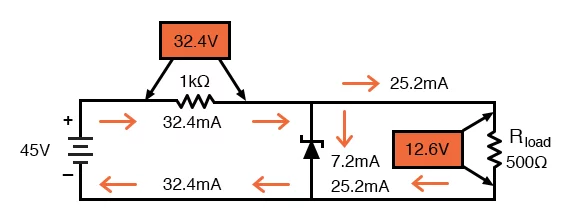# Zener Diode Power Rating Calculation

## A Zener diode is an electronic component used in DC voltage regulator circuits. The power rating of a Zener diode indicates the maximum power that the diode can safely dissipate.  In the formula below, power equals the voltage multiplied by the current. IZM is the maximum current that can flow through the diode, and PZM is the diode’s maximum power dissipation, and VZ is the Zener voltage.

Inputs
Vsource
Volt
Vout
Volt
Imax
mA

Output
Zener diode power rating
Resistance value
Resistance power rating

Knowing the maximum current that can flow through the Zener diode is important, because exceeding its power rating can damage or destroy the component.

### Equation

Formula —

$$IZM = PZM/VZ$$

Example: If a Zener diode has a voltage of 5.1V and a power rating of 1W, what is the maximum current that can flow through the diode?

IZM = 1W/5.1V = 196 mA

### Application

Zener diodes can be used in simple voltage regulator circuits, such as the one shown below. Note that the series resistor limits the current through the Zener diode.

###